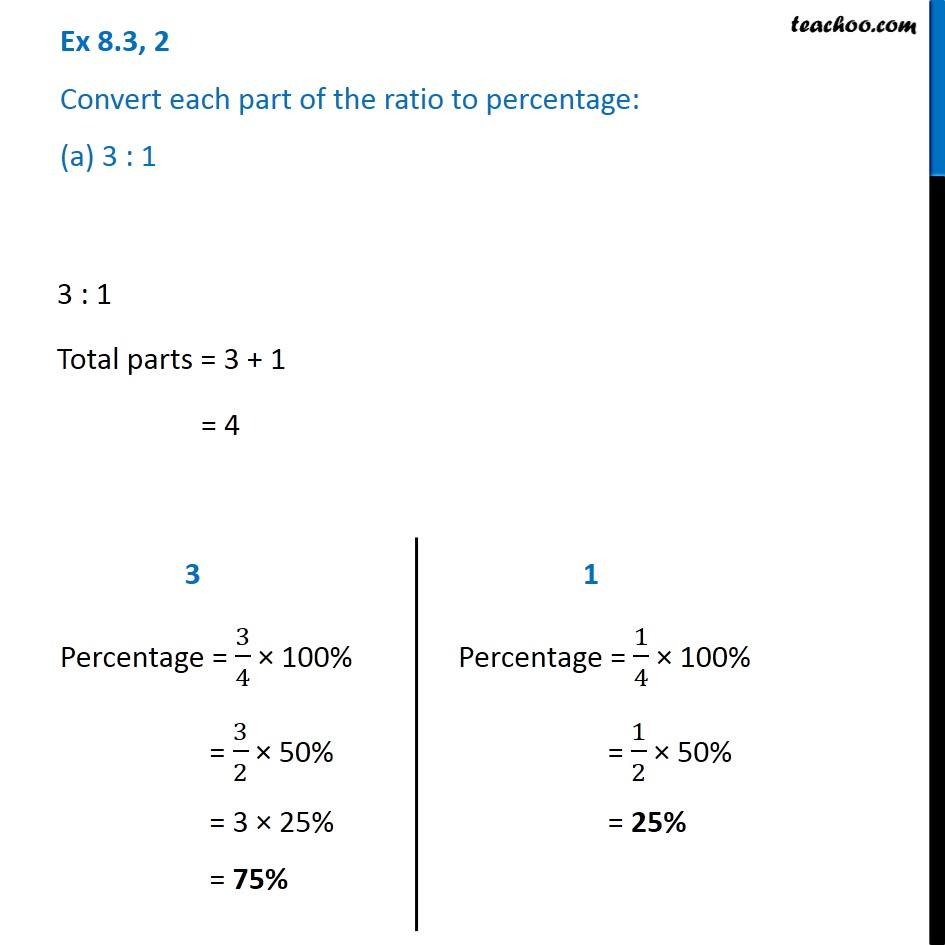Ratios to percentage

Chapter 8 Class 7 Comparing Quantities
Concept wiseIntroducing your new favourite teacher - Teachoo Black, at only ₹83 per month

### Transcript

Ex 8.3, 2 Convert each part of the ratio to percentage: (a) 3 : 1 3 : 1 Total parts = 3 + 1 = 4 3 Percentage = 3/4 × 100% = 3/2 × 50% = 3 × 25% = 75% 1 Percentage = 1/4 × 100% = 1/2 × 50% = 25%